# A Language Speed Test Comparing Embedded Linux Boards

October 2, 2015, updated October 3, 2015

This is a fairly interesting test because of its simplicity when implementing across many different programming languages- even if it is not tailored specifically to idioms and abstractions provided by those languages. The test referenced above directly compares programming language speeds and this one instead compares those programming language speeds on different embedded Linux boards. This is not a test to optimize the languages, the code, or the boards to be as fast as possible. Instead it is intended to run the same exact code using the same runtime version of a language on different embedded Linux boards in as identical an environment as possible.

The test code is very simple. When given a number n, the program simply counts the number of prime numbers found up to and including n. The test code is implemented in the following languages:

• C (gcc using -O2 optimization)
• Python 2.7.10 (using -O optimization)
• Python 2.7.10 (Compiled) (using -O optimization)
• Ruby 2.2.2p95
• Lua 5.1.5
• PHP 5.6.12
• Javascript (nodejs v0.10.40)

on the following boards:

The final result is enlightening. Here is the comparison of counting prime numbers up to 224. The runtimes here are significant enough to get a good comparison across boards in each of the languages tested. Note that lower times mean faster.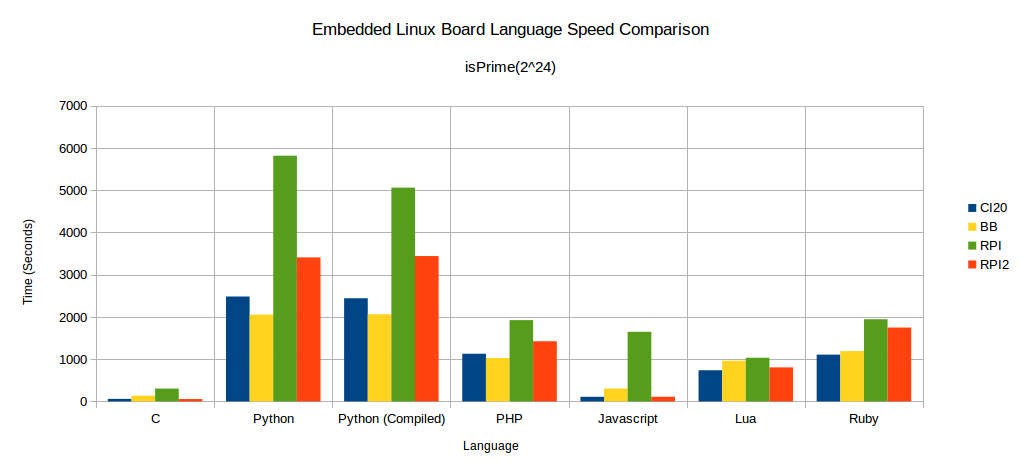If a lower value of n is charted, where n is 212, this puts far more weight on startup and initialization times of the test programs.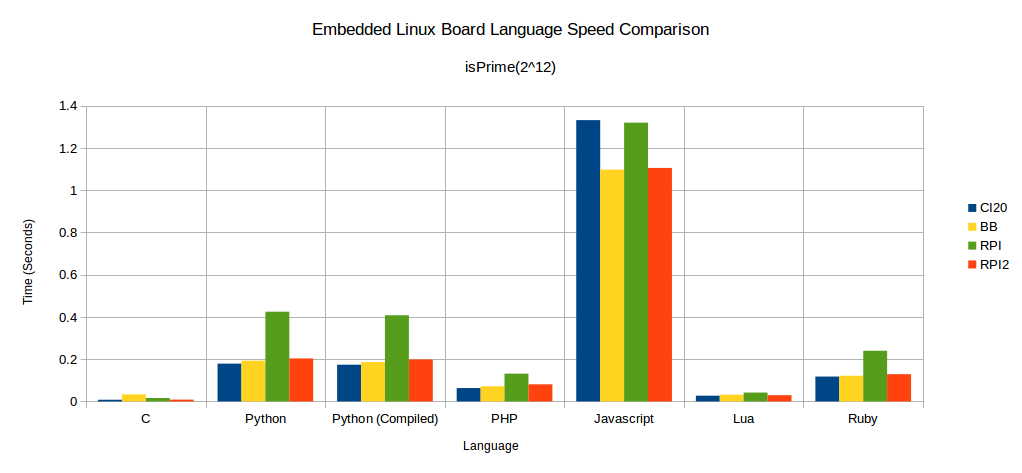Below are charts of the raw and complete data for each of the boards. The full tests are run with n being from 212 to 224.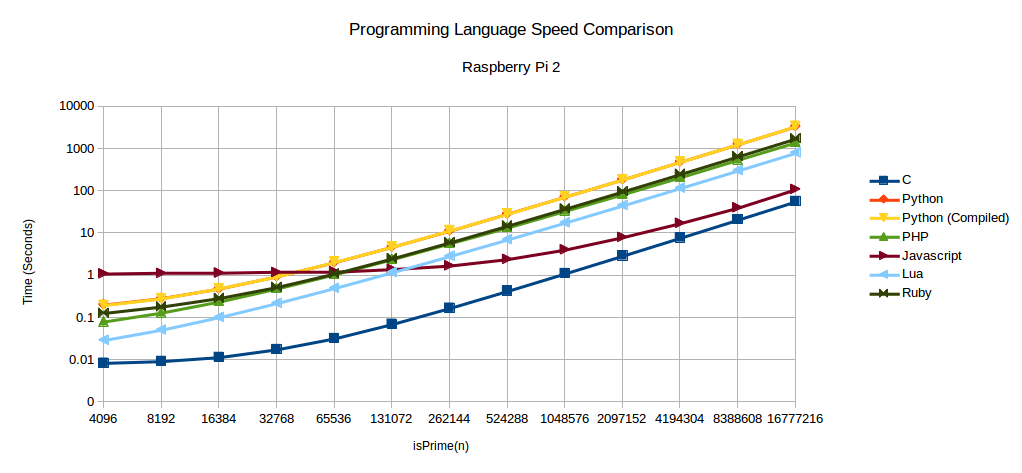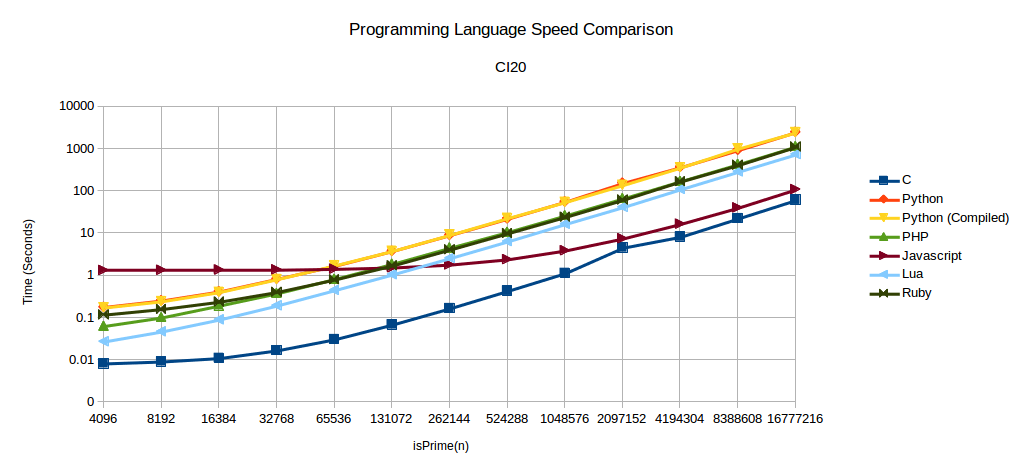Do you see that drop in there in the BeagleBone Black C language data? All data was collected twice and that abnormality existed in both of them. I went back and manually ran the test and the same thing happened. Just wanted to point out this is not a mistake and I am wondering what happens there.

This basic test is enough to heat up every single processor enough to be fairly warm to the touch. The BeagleBone black got the hottest with a 142F reading on the surface of the SoC.

The test consisted of building the same version of buildroot default configurations for all boards. This included creating bootloaders, a kernel, and a rootfs containing the languages and test code on a uSD/SD card. These were all setup in a headless configuration with the only interface being a serial console. It turns out, this was quite a time consuming processing to get setup for each board.

### CI20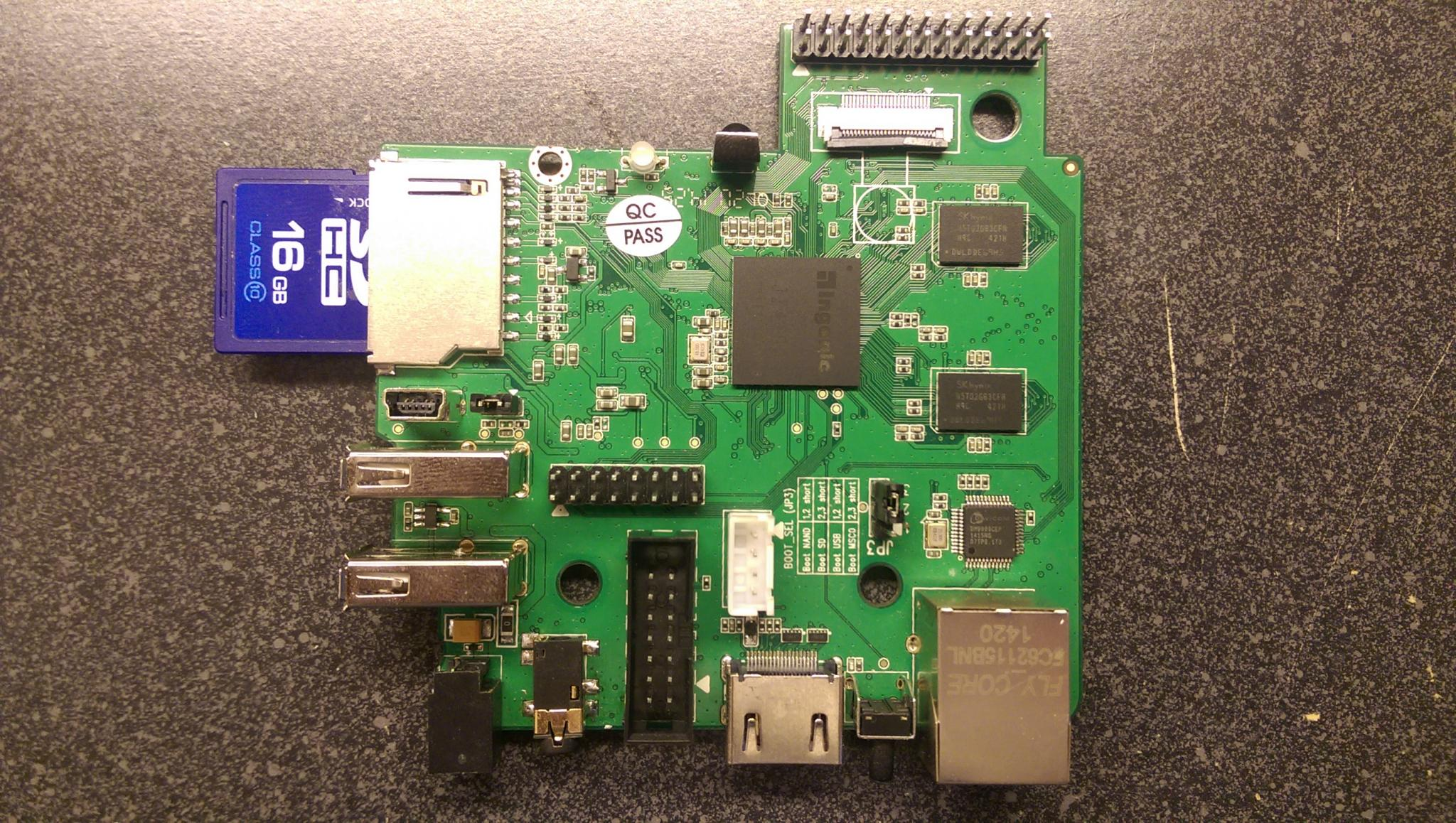### BeagleBone Black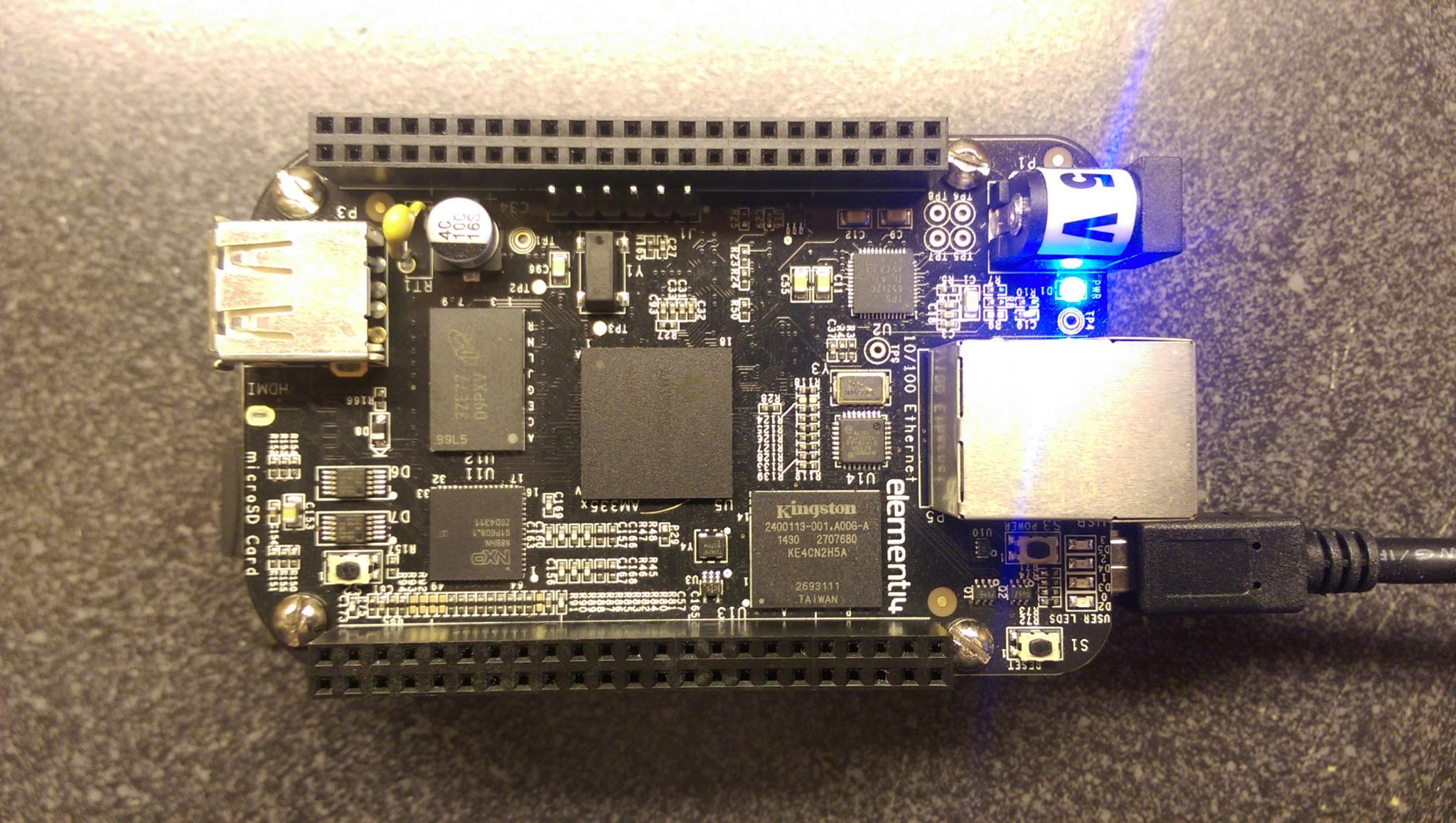### Raspberry Pi 2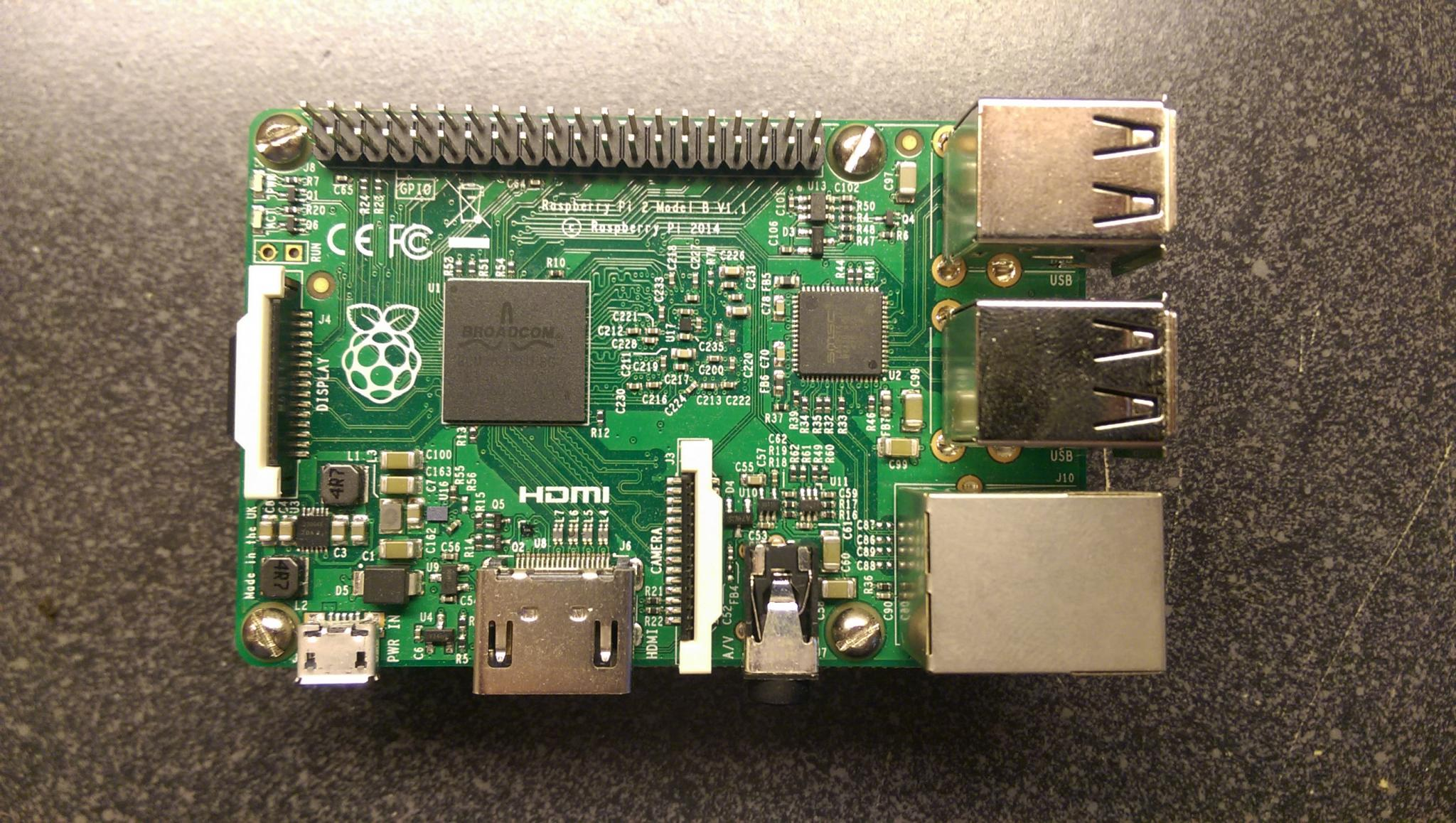### Raspberry Pi Model BThe following are the actual test programs written in the various languages to compute the number of prime numbers given an argument of n.

### Lua

```function isPrime(n)
if (n < 2) then
return false
elseif (n == 2) then
return true
elseif (n % 2 == 0) then
return false
end

for i = 3, math.sqrt(n), 2 do
if (n % i == 0) then
return false
end
end

return true
end

local noPrimes = 0
local limit = tonumber(arg)
for n = 0, limit, 1 do
if isPrime(n) then
noPrimes = noPrimes + 1
end
end

print(string.format("pi(%d)",noPrimes))```

### C

```#include <stdio.h>
#include <math.h>
#include <stdlib.h>

int isPrime(int n) {
if (n < 2)
return 0;
else if (n == 2)
return 1;
else if (n % 2 == 0)
return 0;

int upperLimit = sqrt(n);
int i = 3;
while (i <= upperLimit) {
if (n % i == 0)
return 0;
i += 2;
}
return 1;
}

int main(int argc, char** argv) {
int noPrimes = 0;
int limit = atoi(argv);
for (int n = 0; n <= limit; n++) {
if (isPrime(n))
noPrimes ++;
}
printf("pi(%d)\n", noPrimes);
return 0;
}```

### Javascript

```function isPrime(n) {
if (n < 2)
return false;
else if (n == 2)
return true;
else if (n % 2 == 0)
return false;
var uL = Math.sqrt(n) | 0, i = 3;
while (i <= uL) {
if (n % i == 0)
return false;
i += 2;
}
return true;
}

var noPrimes = 0;
var limit = process.argv;

for (var i = 0; i <= limit; i++) {
if (isPrime(i))
noPrimes++;
}

console.log("pi(" + noPrimes + ")");```

### PHP

```<?php
function isPrime(\$n) {
if (\$n < 2)
return false;
elseif (\$n == 2)
return true;
elseif (\$n % 2 == 0)
return false;
\$ul = sqrt(\$n);
\$i = 3;
while (\$i <= \$ul) {
if (\$n % \$i == 0)
return false;
\$i+=2;
}
return true;
}

\$noPrimes = \$i = 0;
\$limit = \$argv;

while (\$i <= \$limit) {
if (isPrime(\$i))
\$noPrimes++;
\$i++;
}

printf("pi(%d)\n", \$noPrimes);
?>```

### Python

```from math import sqrt
import sys

def isPrime(n):
if (n < 2): return False
elif (n == 2): return True
elif (n % 2 == 0): return False

upper_limit = int(sqrt(n))
i = 3
while (i <= upper_limit):
if (n % i == 0): return False
i += 2

return True

lim = int(sys.argv)
no_primes = 0
n = 0

while (n <= lim):
if (isPrime(n)): no_primes += 1
n += 1

print ("pi(%d)" % (no_primes))```

### Ruby

```def isPrime n
return false if n < 2
return true if n == 2
return false if n % 2 == 0

upper_lim = (Math.sqrt n.to_f).to_i
i = 3
while i<= upper_lim
return false if n % i == 0
i+=2
end

true
end

lim=(ARGV).to_i
no_primes = 0

i = 0
while i <= lim
no_primes += 1 if isPrime i
i+=1
end
puts "pi(#{no_primes})"```
Update 10-2-15: Updated charts for individual boards to have a logarithmic scale so the data can be seen better.

## 8 Comments

Comment October 2, 2015 by Alessandro Stamatto
Great work. Very nice article! Javascript for math seems to have an insane JIT, always so fast on those math benchmarks @o@ I posted it on the programming reddit ( https://www.reddit.com/r/programming/comments/3n6qk1/a_language_speed_test_on_embedded_linux_boards/ ) (And there are some suggestions/questions there)
Comment October 2, 2015 by Angel Aray
It would be interesting to see Go included in the list.
Comment October 2, 2015 by Isaac Gouy
fyi Tiny tiny prime sieve programs are available in the old benchmarks game code archive for a variety of languages. See nsieve and sieve: https://alioth.debian.org/scm/viewvc.php/shootout/bench/nsieve/?root=shootout&hideattic=0 https://alioth.debian.org/scm/viewvc.php/shootout/bench/sieve/?root=shootout&hideattic=0
Comment October 2, 2015 by Isaac Gouy
>>The test referenced above directly compares programming language speeds and this one instead compares those programming language speeds on different embedded Linux boards.<< Except that as soon as you show a chart with different programming language implementations, your readers **will** directly compare the programming language speeds. For example -- "Wow, really surprised by the javascript results there, did not expect it to be so close to c."
Comment October 2, 2015 by anonymous
Angel: How would go work on MIPS boards? AFAIK it only has x86/x64/arm support.
Comment October 3, 2015 by digitalpeer
@Alessandro Javascript is obviously the surprise here (for me anyway), but it's worth noting nodejs has JIT unlike the other interpreted languages. It goes without saying some of the others have JIT capable runtimes that are not used here. Thanks for posting to /r/programming. The feedback has been awesome as usual. @Angel While Go, Rust, and friends are the hot "new" languages, this also means that support for them is not, well, mature. In some ways, embedded lags what happens in PC/Server world here. For example, these languages are not part of Buildroot. However, I'm working on it. @Isaac Point well taken. While my intent was to compare embedded Linux boards, data is data. As long as the parameters of the test are understood, people can use it to come up with whatever conclusions they want. I find this sort of data interesting. This gives me the opportunity to stand back and see the bigger picture.
Comment October 3, 2015 by anonymous
What is "compiled" Python? If you're just talking about generating the .pyo files, that takes a constant few milliseconds and doesn't really add any new information to the discussion.
Comment February 13, 2016 by Thom
Would love to see Erlang in this test. It's fault tolerance is a good companion to embedded devices.